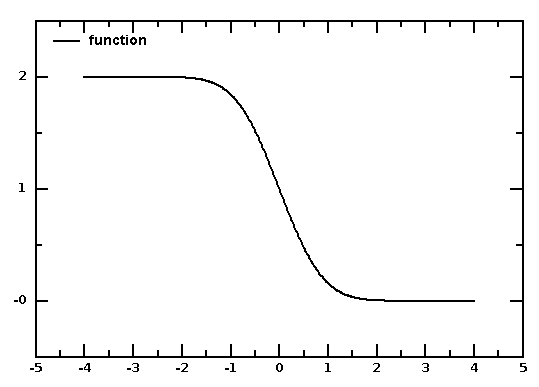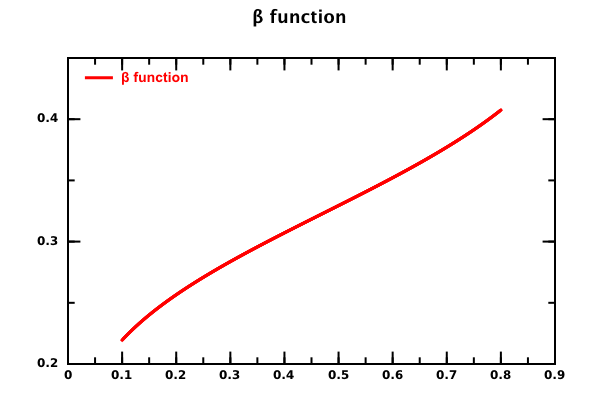# DMelt:Math/4 Special Functions

Limitted access. First login to DataMelt if you are a full DataMelt member. Then login to HandWiki as a user.

# Special functions

The simplest way to create complicated functions including special function is to call external Java libraries. You can use any Java library in the function definitions, mixing freely Java and Python code (or any other scripting language). Here is a small example that creates 1-erf(x) function fromApache math3.special package. to define the "erf" function.

from  jhplot import *
from org.apache.commons.math3.special.Erf import *

class MyFunc(FNon):
def value(self, x):
return 1-erf(x)
c1 = HPlot()
c1.visible();   c1.setAutoRange()
pl = MyFunc("function",1,0)
f1=F1D(pl,-4,4)
c1.draw(f1)

The function "erf" is taken fromErf Java class. Here "MyFunc" is a class that defines a new function (1-erf) and returns its values. x defines "x" (0 means one-dimensional function). You can also define functions in multiple dimensions using x, x, x etc. notations.

The output of this script is shown in this plot:The above approach works only for Jython/Python codding. A more general approach is to call Java directly and extend the classjhplot.FNon that is a pure-Java approach, as in the above example. In this case you can create not only Python scripts, but also write code in pure Java, or mix Java, Groovy and JRuby.

Look at the section Non-parametric functions for more details.

As you can see, you can mix Java, Jython and your custom code. A beta function created with the above code is shown here:Here is another example: let us plot a Crystal Ball function as defined Crystal_Ball_function. We will use the Python language to create this function, and then we will plot it:

with the output shown below:

Not allowed to read dmelt 20415788 : Not datamelt.org URL!

## List of Java libraries for special functions

Here is a short list of special functions supported by DataMelt:

In addition, DataMelt supports distribution functions: